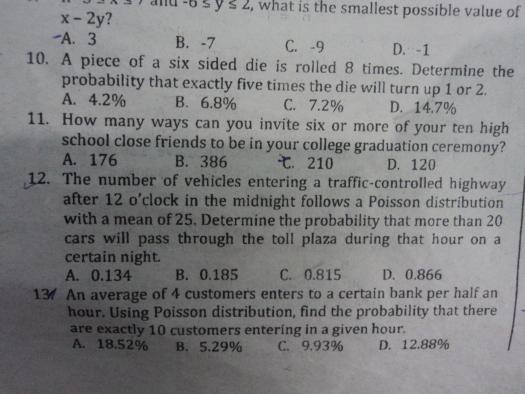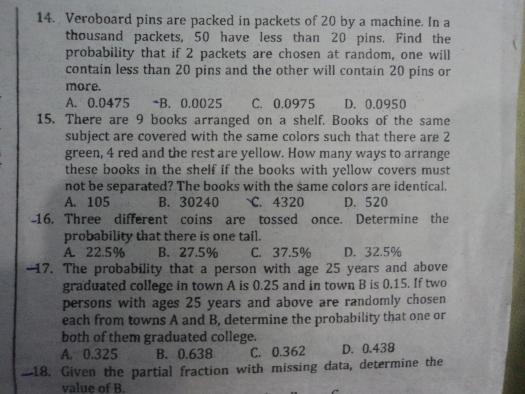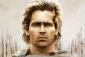# probability: A six-sided die is rolled 8 times [ Multiple Problems - LOCKED ]### Re: probability

guys kindly show me your solutions as well. tnx### Re: probability

#10 A piece of six sided die is rolled 8 times. Determine the probability that exactly five times the die will turn up 1 or 2.

One possible outcome is $$x_1, ~ (1 \text{ or } 2), ~ (1 \text{ or } 2), ~ x_2, ~ (1 \text{ or } 2), ~ x_3, ~ (1 \text{ or } 2), ~ (1 \text{ or } 2)$$

Where x1, x2, and x3 are outcomes that neither 1 nor 2. The probability for the above outcome to happen is
$$\left( \dfrac{4}{6} \right)^3 \left( \dfrac{2}{6} \right)^5$$

The above outcome may happen or it can happen in different ways provided there are five (1 or 2)s and three non (1 or 2)s. The keyword here is "or", hence, the total number of ways we can roll a die 8 times so that (1 or 2) will appear exactly 5 times is
$$\binom{8}{5}$$

The probability that exactly 5 times the die will turn up 1 or 2 is
$$\binom{8}{5} \left( \dfrac{4}{6} \right)^3 \left( \dfrac{2}{6} \right)^5$$

Note: $\displaystyle \binom{8}{5} = \dfrac{8!}{5!(8 - 5)!}$### Using Binomial

Using Binomial
P = nCx px qn-x
P = 8C5 (1/6)5 (5/6)8-5
P = 875 / 209,952### salamat sir. ung #14 po, di

salamat sir. ung #14 po, di ko talaga ma gets. :(

### Problem 14:

Problem 14:
P1(getting <20pins)= 50/1000
P2(getting >20pins)= 950/1000

2 packets are chosen in random, favorable outcomes for one contains lesser than 20 pins and the other more than 20 pins is
(<20pins, >20pins) or (>20pins, <20pins),
Thus,
P1*P2 + P2*P1= 0.095